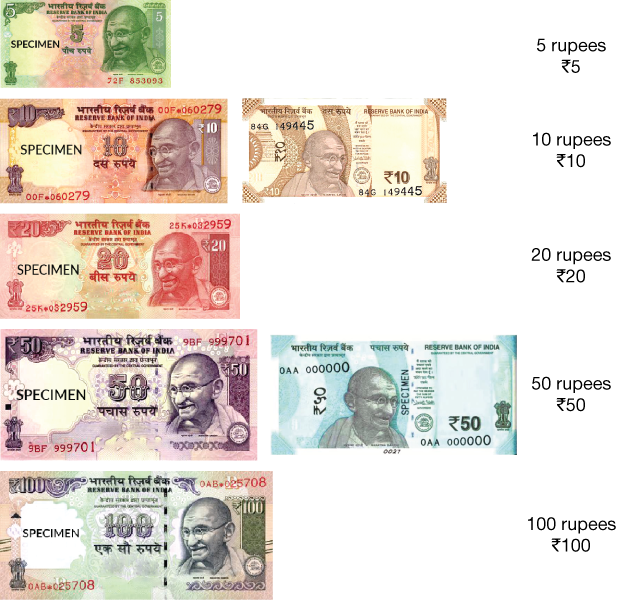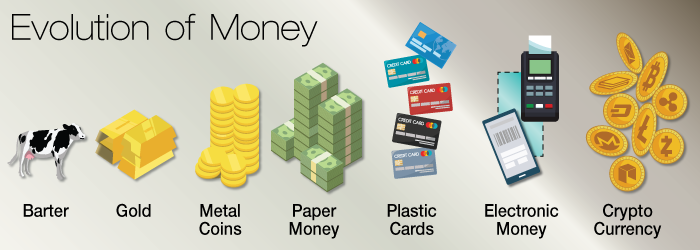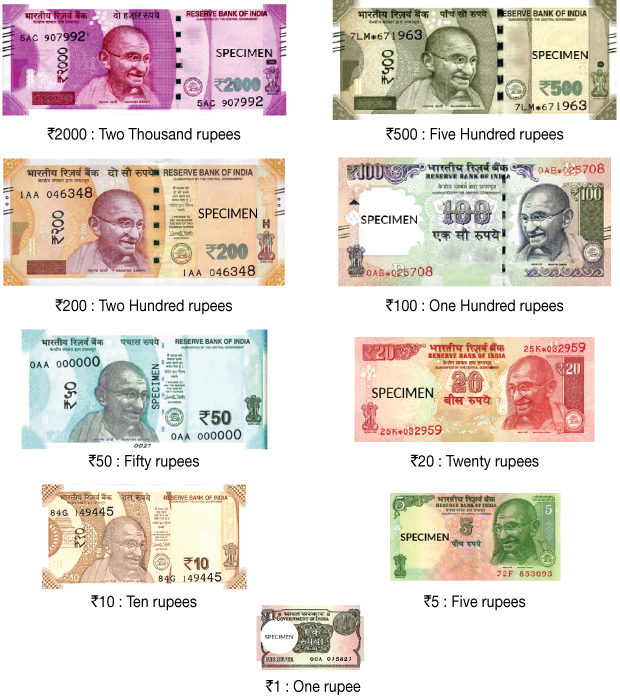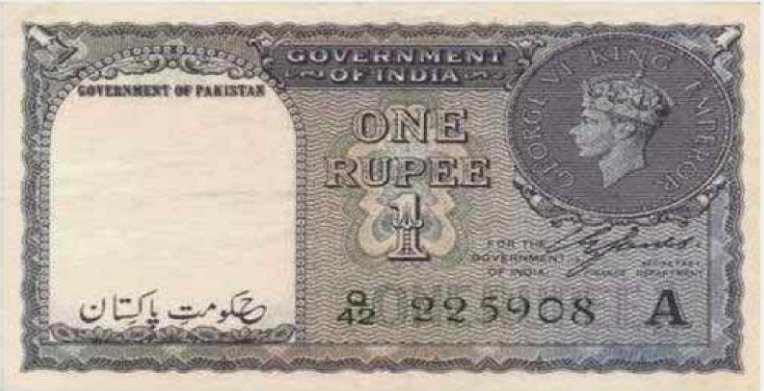# Identifying Denominations

Go back to  'Money'

## Introduction to Identifying Denominations

When you pay your school fees, you use money. When you need to buy your tiffin during the lunch break at school, you need money. If you need to buy a notebook in the evening, you need money. For everything we think of doing throughout the day, there is a chance of money being involved. Maybe your parents’ love for you is one of the few things in this world that will never involve money.

This page is devoted to the understanding of how to identify denominations.## The Big Idea: What are the denominations of money?

Long back, the common currency for any kind of commerce was called the barter system. So, someone who had more rice than he needed would look for someone who had salt to give. So, a sack of rice could be exchange for, say, 5 sacks of salt. But modern currency has moved away from such exchanges and uses paper currency or coins for all commercial transactions. But we need to be able to distinguish different sizes of coins and different sizes and colours of paper, depending on what their denomination is. So, denomination of a currency notes or coin denotes the value of the note or the coin.How do we understand the denominations? You must recall how we learnt about numbers and their place values of numbers. So, we have units, tens, thousands, ten thousands, lakhs and so on when it comes to numbers. The denominations of numbers are counted in the same way. Currency notes or coins can be in units, or in tens, or hundreds, or thousands. So, if you have a thousand rupees, it is equivalent to the 1000 place value.

### The different denominations we use

Let us take a look at the image below, which tells us what are the different denominations of currency notes we have in India at present. These are our current denominations:

\begin{align} 2000 &\text{ : Two Thousand rupees}\\ 500 &\text{ : Five Hundred rupees}\\ 200 & \text{ : Two Hundred rupees}\\ 100 &\text{ : One Hundred rupees}\\ 50 & \text{ : Fifty rupees}\\ 20 &\text{ : Twenty rupees}\\ 10 &\text{ : Ten rupees}\\ 5 &\text{ : Five rupees}\\ 1 & \text{ : One rupee} \end{align}Re.1, Rs.10, Rs.100 are all similar to the place values of Units, Tens and Hundreds respectively. Using these basics, we can build up to the other denominations. For example, 4 notes of Rs.50 can be equivalent to 1 note of Rs.200. But that same Rs.200 can also be equivalent to two notes of Rs.100. So while currency note denominations are similar to the place value of small or big numbers, each bigger denomination of currency can be composed of more than one combination of smaller denominations. We will look at that in detail when we discuss decomposition of money.

## The Importance of Understanding Denominations

Let us say you ask your father for some chocolates and cookies, and he gives you a hundred rupee note. You buy 2 chocolate bars of Rs.10 each, and you buy 3 cookies of Rs.5 each. Your total expense = (2 x 10) + (3 x 5) = 20 + 15 = 35.

You have done this math and handed over the hundred rupee note to the shopkeeper. You also do the math in your head and find out that you will get change of Rs.65 back. But what are the denominations you will get your money back in? There are several ways, and a few are listed here:

1. You get one note of Rs.50, one of Rs.10, and one of Rs.5. So the total = 50 + 10 + 5 = 65.
2. You get two notes of Rs.20, you get two of Rs.10, and one of Rs.5. So the total = (2 x 20) + (2 x 10) + (1 x 5) = 40 + 20 + 5 = Rs.65.
3. You could also get six notes of Rs.10 and one of Rs.5. So the total = (6 x 10) + (1 x 5) = 60 + 5 = Rs.65

For a two-digit value of money there are so many ways, so just imagine how many combinations of currency notes and coins would be there in larger numbers. That is why it is very important to understand denominations.

### An Interesting Story

Before we end, here is an interesting story about Indian currency notes. When India and Pakistan became two different countries in 1947, Pakistan did not have the knowhow to print its own currency yet. So, it continued to use Indian currency notes with ‘Government of Pakistan’ stamped on them.Money
Money
Money
Money
Money
Money
Money